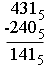SEARCH HOMEMath Central Quandaries & QueriesQuestion from Nikki, a student: can you help me how to do subtraction in base 5 notation. example is 3434 - 51? how do you do this in base 5? can you please explain how you do this & the answer as i have no idea. Thank you kindly NikkiHi Nikki,

If you are using base five notation to express numbers then the only symbols you have for digits are 0, 1, 2, 3, and 4. Thus 51 can't be a base five number.

You should first look at my note to Jana on adding using base five notation. Subtraction is straightforward of you are always subtracting a smaller digit from a larger digit. The challenge is to deal with borrowing. Let's look at a base 10 problem first.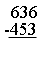Starting in the first column 6 - 3 = 3 but in the next column you need to borrow from the third column. Since this is base ten notation you are borrowing ten so the 6 in the third column becomes 5 and adding ten to 3 you have thirteen in the second column.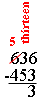5 from 13 is 8 and 4 from 5 is 1 so the result is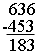Now let's try a base 5 problem.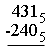As in the base ten problem the first column is easy, 1 - 0 = 1. In the second column you need to borrow from the third column. Since the numbers are written in base five notation you are borrowing five so the 4 in the third column becomes 3 and adding five to 3 gives you eight in the second column.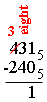4 from eight is 4 and 2 from 3 is 1 so the result is